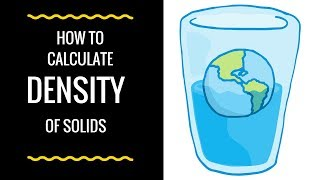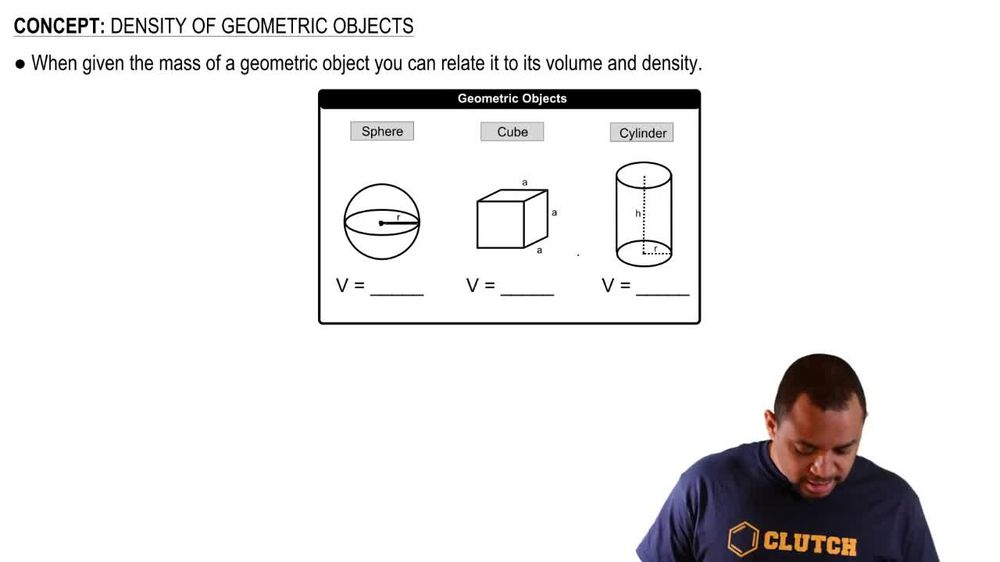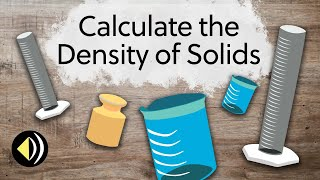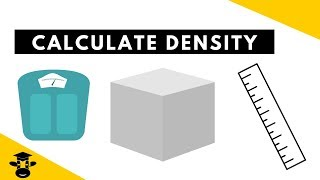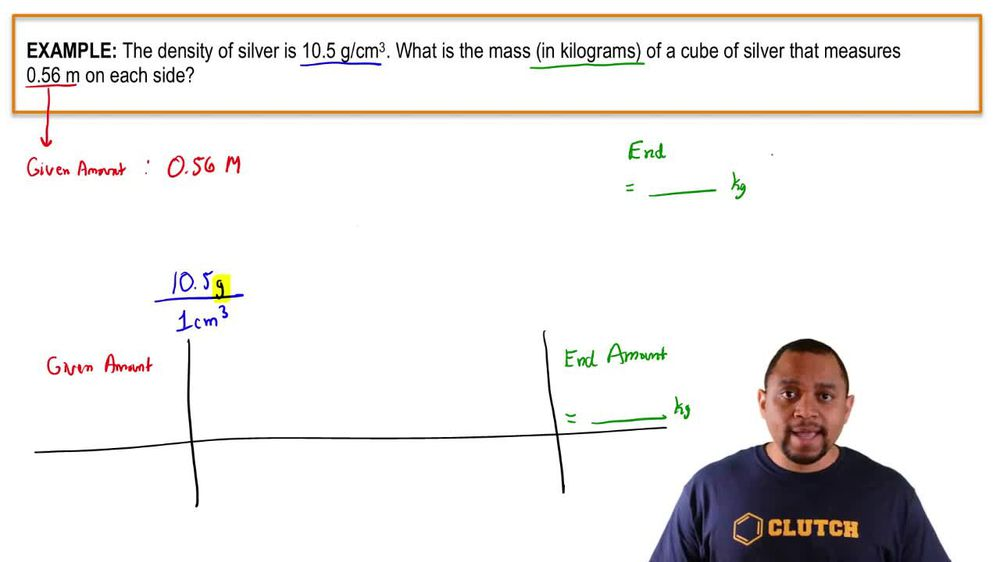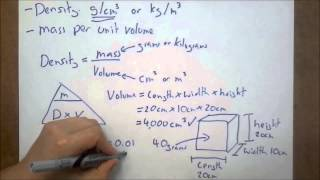Start typing, then use the up and down arrows to select an option from the list.
1. 1. Intro to General Chemistry2. Density of Geometric Objects
Problem

# An 8.894 g block of aluminum was pressed into a thin square of foil with 36.5 cm edge lengths. (a) If the density of Al is 2.699 g>cm3, how thick is the foil in centimeters?

Relevant Solution4m
Play a video:
Hi everyone. This problem reads a zinc slab weighing 23.5 g was shaped into a square sheet with an edge length of 21. centimeters. If the density of zinc is 7.13 g per cubic centimeter, determined the thickness of the sheet in centimeters. Okay, so this is what we want to solve for. So let's go ahead and get started. So we know we have a square sheet with an edge length of 21.5 cm. And in order for us to determine the thickness, we need to know that volume is equal to length times with times height. Okay, for a square sheet which we're told here for something that is a square sheet, the length is going to equal the width. Okay, and here height is equal to thickness. So that means in order for us to solve for the height, we need to rearrange this equation. Okay, so let's put this here length is equal to width, but height is equal to thickness. So we need to isolate our height variable so we'll divide both sides by length times width and we get height is equal to volume over length times with Okay, So for this problem, we already know what the length and width are because it tells us that it is a square sheet with an edge length of 21.5 cm. So that means our length and our with our both 21.5. So that means our denominator for here is going to be 21.5 cm Times 21.5 cm. All right. So that leaves us with calculating volume. The volume was not given in the problem, but we know what the weight is. Okay. We know we have 23 five g. And so now we need to figure out what is our volume starting from grams? The weight. Okay? So let's go ahead and write that here. So we know we have 23.50 g. And our goal is to go from grams two volume, which is cubic centimeters. Okay? The way that we can go from grams to cubic volume or from mass to volume is if we know the density and the problem we do know the density, we're told the density is 7.13 g per cubic centimeter. So the way we set this up for it to cancel properly is we put the grams on the bottom, so 7.13 g and we put cubic centimeters at the top. All right. So we make sure our units cancel our units of grams cancel. And so now we're going to take 23.5 and divide it by 7.13. When we do this, we get a volume of 3.2959 cubic centimeters. So this is the volume that we need to plug into our height equation. So we have 3. cubic centimeters. Okay? So now we have everything that we need to solve for height. So let's go ahead and do this calculation will take 3. cubic centimeters and divided by 21.5 centimeters squared. Okay, So we get a final answer of 7. times 10 to the negative three centimeters. So this is going to be the thickness of the sheet and centimeters. Alright, and that is our final answer. That's it for this problem. I hope this was helpful.